2017年01月15日

2017年01月15日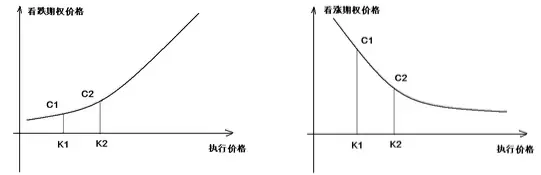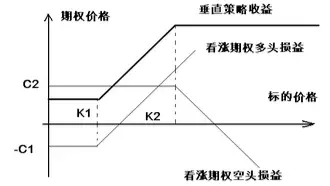1、买入蝶式套利（Long Butterfly）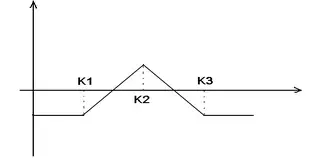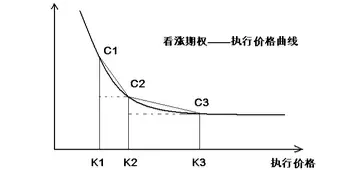(C1-C2)/(K2-K1)>(C2-C3)/(K3-K2)

(K3-K2)/(K3-K1)*C1+ (K2-K1)/(K3-K1)*C3>C2

C2-(K3-K2)/(K3-K1)*C1+ (K2-K1)/(K3-K1)*C3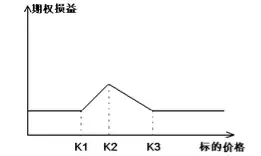2、卖出蝶式套利（Short Butterfly）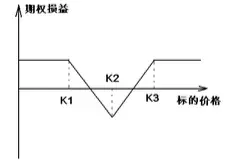(K3-K2)/(K3-K1)*C1+ (K2-K1)/(K3-K1)*C3>C2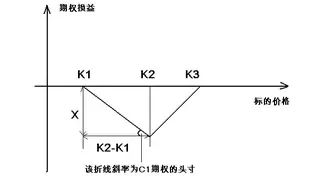(K3-K2)/(K3-K1)*C1+ (K2-K1)/(K3-K1)*C3-C2>(K3-K2)/(K3-K1)*(K2-K1)

1、买入飞鹰式套利（Long Condor）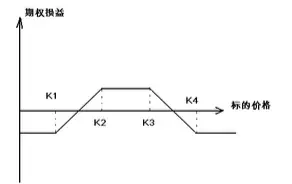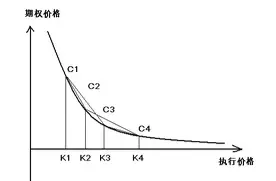(K4-K2)/(K4-K1)*C1+ (K2-K1)/(K4-K1)*C4>C2

(K4-K3)/(K4-K1)*C1+ (K3-K1)/(K4-K1)*C4>C3

(2K4-K3-K2)/(K4-K1)*C1+ (K2+K3-2K1)/(K4-K1)*C4>C2+C3

(2K4-K3-K2)/(K4-K1)*C1+ (K2+K3-2K1)/(K4-K1)*C4< P>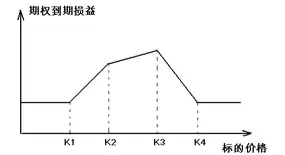2、卖出飞鹰式套利（ShortCondor）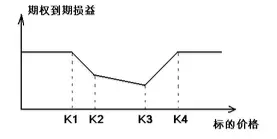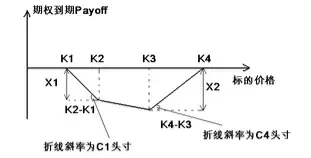X1=（K2- K1）* C1的头寸，X2=（K2- K1）* C4的头寸。

(2K4-K3-K2)/(K4-K1)*C1+ (K2+K3-2K1)/(K4-K1)*C4>C2+C3

(2K4-K3-K2)/(K4-K1)*C1+ (K2+K3-2K1)/(K4-K1)*C4-（C2+C3）>0

(2K4-K3-K2)/(K4-K1)*C1+ (K2+K3-2K1)/(K4-K1)*C4-（C2+C3）>max（X1,X2）

X2 = (K4-K3)*(K2+K3-2K1)/(K4-K1)1、买卖权平价套利

C-P=S-Ke-rT，

2、箱式套利会员收藏